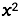# Foiling the Parrot Beak

## OBJECTIVE: To learn how to multiply two binomials

In a previous lesson, you learned how to multiply a monomial by a polynomial and divide a polynomial by a monomial. Sharing the Booty: https://www.geogebra.org/m/x8adhm2g In this lesson, you'll learn how to multiply two binomials using the Parrot Beak Method or the FOIL Method.

## TRY IT YOURSELF ...

The applet below will drill you on multiplying two binomials. On a separate sheet of paper, multiply the binomials you see on the applet. Be sure to simplify your expression afterward. Enter this expression into the input box provided. (Enter "x^2" to show). If you enter the correct expression, you'll see a big "CORRECT !!!" sign appear. Otherwise, you'll have to redo your multiplication. Repeat as many times as needed to master the concept.
﻿Below is a set of problems that require you to find the product of two binomials. First, solve the problems on scratch paper. Then check the Answer Box below for the correct answers.

Check out the answers to the above problems here.

## TODAY you learned how to multiply two binomials.

In future lessons, you'll learn how to multiply other types of polynomials. Did you ENJOY your lesson today?